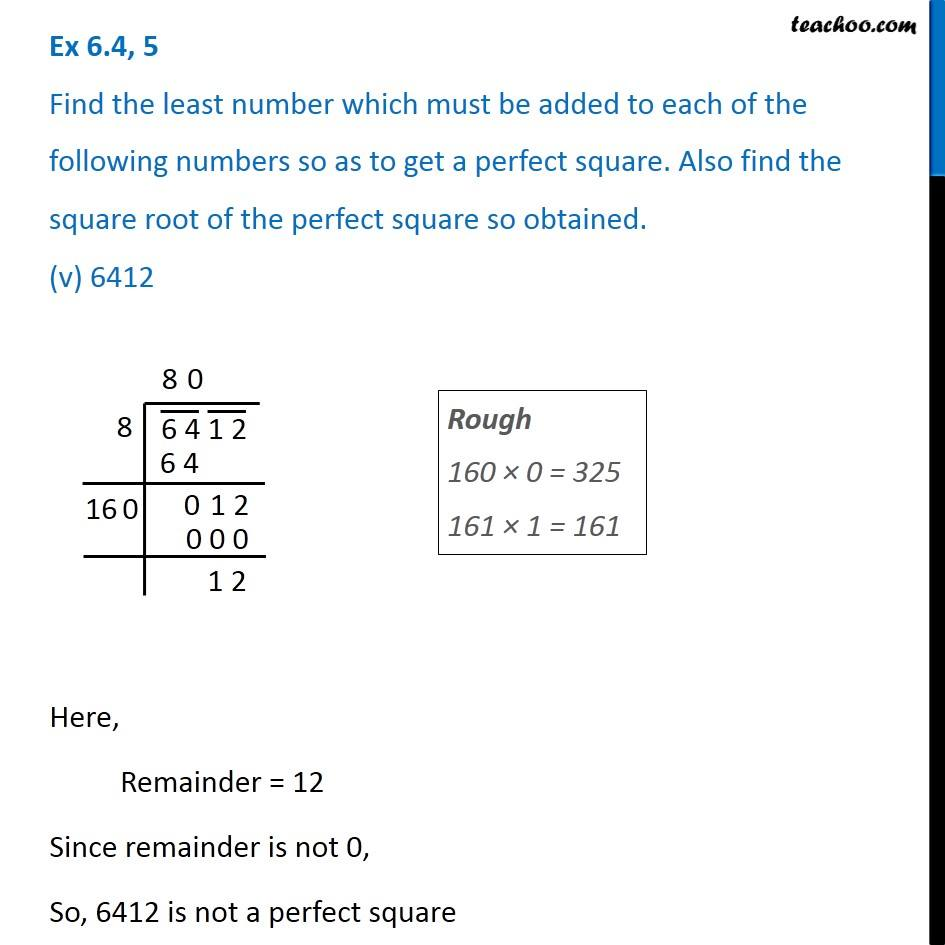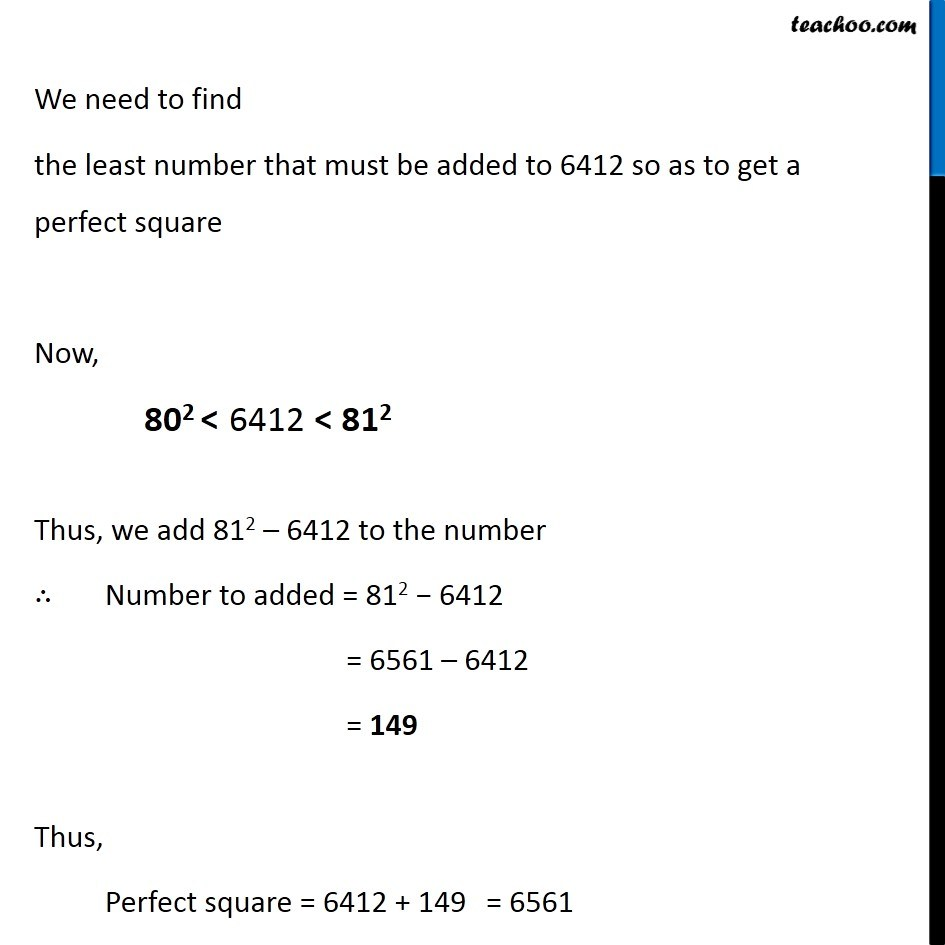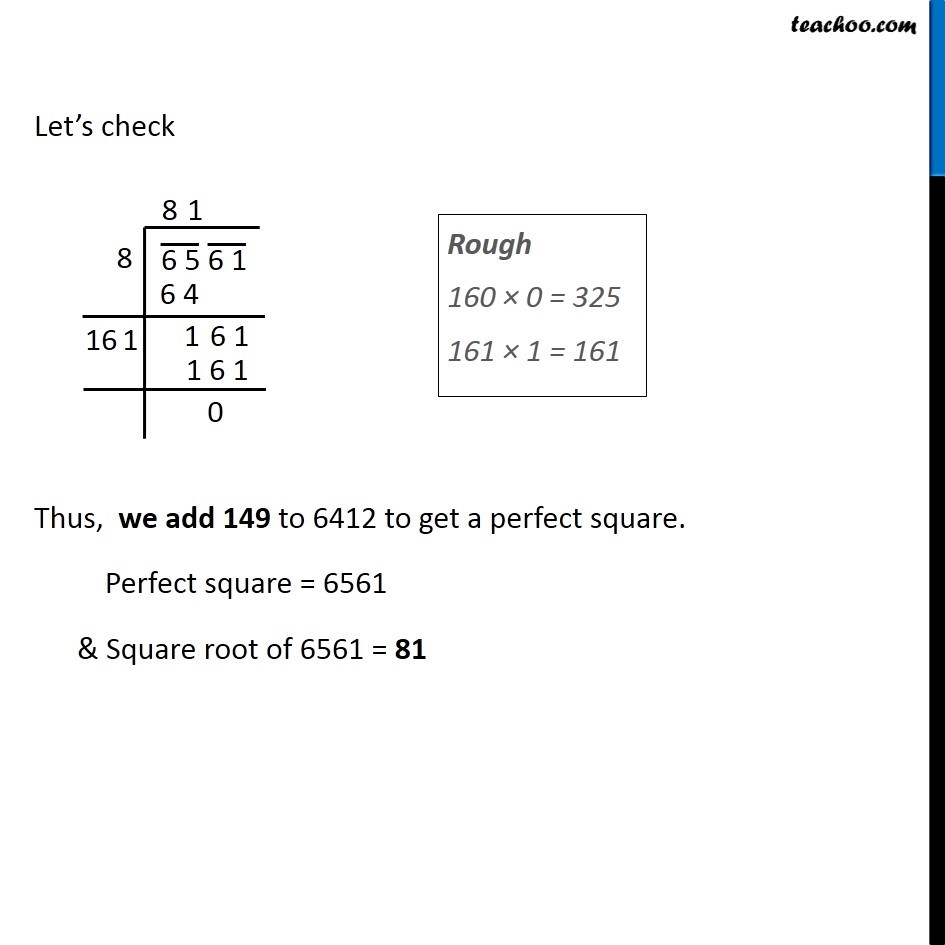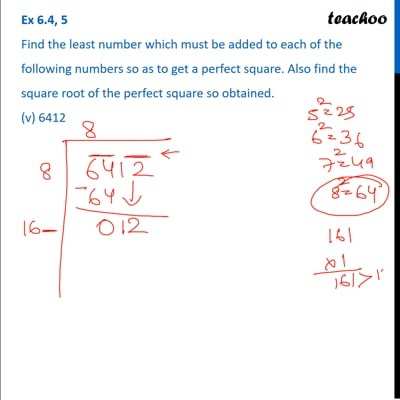Ex 5.4

Chapter 5 Class 8 Squares and Square Roots
Serial order wiseThis video is only available for Teachoo black users

Learn in your speed, with individual attention - Teachoo Maths 1-on-1 Class

### Transcript

Ex 5.4, 5 Find the least number which must be added to each of the following numbers so as to get a perfect square. Also find the square root of the perfect square so obtained. (v) 6412Rough 160 × 0 = 325 161 × 1 = 161 Here, Remainder = 12 Since remainder is not 0, So, 6412 is not a perfect square We need to find the least number that must be added to 6412 so as to get a perfect square Now, Thus, we add 812 – 6412 to the number ∴ Number to added = 812 − 6412 = 6561 – 6412 = 149 Thus, Perfect square = 6412 + 149 Let’s check Thus, we add 149 to 6412 to get a perfect square. Perfect square = 6561 & Square root of 6561 = 81 Rough 160 × 0 = 325 161 × 1 = 161